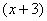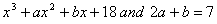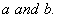# MATHEMATICS — 2004 (Set II — Compartment Outside Delhi)

Except for the following questions, all the remaining questions have been asked in Set I.

Q.3. Ifis a factor of and, findQ. 5. A loan of Rs.91,000 is to be returned in three equal annual instalments. If the rate of interest is 20% per annum, compounded annually, find each annual instalment.

Q. 6. A fan is available for Rs.1,500 cash or for Rs.500 as cash down payment followed by three monthly instalments of Rs.350 each. Find the rate of interest under the instalment plan.

Q. 9. Draw a circle of diameter 8 cm. From a point P, 7cm away from its centre, construct a pair of tangents to the circle. Measure the lengths of the tangent segments.

Q. 11. The monthly incomes of A and B are in the ratio of 4: 3 and their monthly expenditures are in the ratio of 13 : 9. If each saves Rs.1,500 per month, find the monthly income of each.

Q. 16. Find the value of k for which the points with coordinates (3, 2), (4, k) and (5, 3) are collinear.

Q. 19. The following table gives the marks scored by 50 students in a class-test:

 Marks 0-100 100-200 200-300 300-400 400-500 500-600 Number of Student 2 8 12 20 5 3

Q. 23. The annual income of Rekha (aged 35 years) is Rs. 2,70,000 (exclusive of HRA). She contributes Rs. 5,000 per month towards provident fund and pays an annual premium of Rs. 12,000 towards her LIC policy. She pays Rs. 2,500 per month as income tax for the first 11 months. Calculate the income tax payable by her in the last month of the year.

for calculating income tax, use the following:

 (a) Standard Deduction Gross income Deduction (i) Upto Rs.75,000 (ii) From Rs.75,001 to Rs.5,00,000 (iii) More than Rs,5,00,000 40% of gross income Rs.30,000 Rs.20,000 (b) Rates of Taxes Slab Income tax (i) Upto Rs. 50,000 (ii) From Rs. 50,001 to Rs. 60,000 (iii) From Rs. 60,001 to Rs. 1,50,000 (iv) Rs. 1.50,000 and above No tax 10% of the amount exceeding Rs.50,000 Rs.1,000+20% of the amount exceeding Rs.60,000 Rs.19,000+30% of the amount exceeding Rs.1,50,000 (c) Rebate in Tax (i) 20% of the savings subject to a maximum of Rs. 14,000 if the gross income is upto Rs. 1,50,000. (ii)15% of the savings subject to a maximum of Rs. 10,500 if the gross income is from Rs. 1,50,001 to Rs. 5,00,000. (d) Surcharge 10% of the tax payable if theannual income is more than Rs.8,50,000. (e) Rebate for women of less than 65 years Rs. 5,000 over and above other admissible rebates.
Maths 2004 Question Papers Class X
CBSE 2004 Question Papers Class X